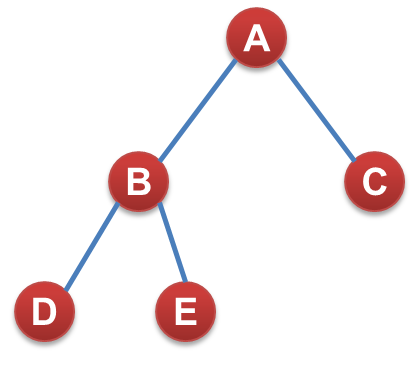# 二叉树的按层遍历法1、把根节点A放入队列，此时队列为：A，队列头指针指向A，也就是队列第一个元素

2、把当前队列头指针所指元素的左右儿子放入队列，即将B C放入队列，此时队列为A B C ，队列头指针向下移一格，此时指向B

3、不断重复2步骤。此时把B的左右儿子取出来放入队尾，队列变为A B C D E，队列头指针后移，指向c，c没有子节点，队列不再延长；

4、结束条件，队列头指针和为指针重合时，输出最后一个元素，算法结束！

1、定义一个二叉树结构

typedef struct binode
{
char data;
struct binode * lchild;
struct binode * rchild;
}BiTree;

2、前序建立二叉树

BiTree * CreatBiTree()
{
BiTree *T;
char data;
fflush(stdin);
scanf("%c",&data);
if(data=='#')T=NULL;
else
{
T=(BiTree *)malloc(sizeof(BiTree));
T->data=data;
T->lchild=CreatBiTree();
T->rchild=CreatBiTree();
}
return T;
}

3、按层遍历二叉树

void Layer_order(BiTree * TNode,BiTree ** F,BiTree ** R)
{

*F=TNode;            //将当前节点放入队列首指针所指位置
printf("%c    ",(*F)->data);
if((*F)->lchild!=NULL)
{
R=R+1;
*R=(*F)->lchild;    //节点的左儿子放入队尾
}
if((*F)->rchild!=NULL)
{
R=R+1;                //首指针向后移动一格
*R=(*F)->rchild;    //节点的右儿子放入队尾
}

if(F!=R)

{

F=F+1;

Layer_order(*F,F,R);//递归

}

}

4、写main函数，建立一个队列，长度1024，存放节点指针（其实就是一个存放指针的数组），这一部分放在main函数中，这个队列是唯一的，不参与递归
BiTree ** F;             //队首指针       指向指针的指针，因为队列数组里的元素全是指针
BiTree ** R;            //队尾指针
BiTree * Queue;//队列数组
F=Queue;
R=Queue;            //开始时队首队尾指针重合

BiTree * root;      //在main函数中建立一个二叉树根的指针

root=CreatBiTree();      //创建树

Layer_order(root,F,R); //按层遍历树

©️2019 CSDN 皮肤主题: 大白 设计师: CSDN官方博客